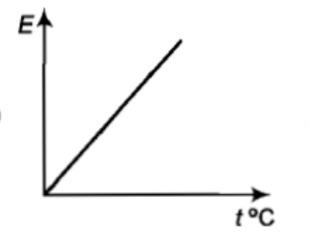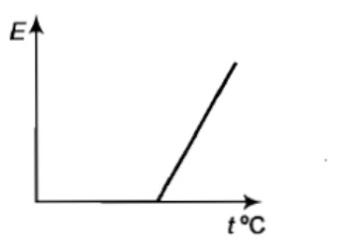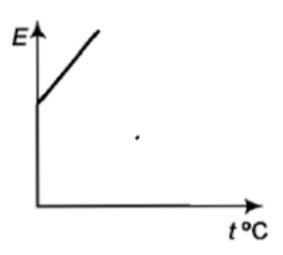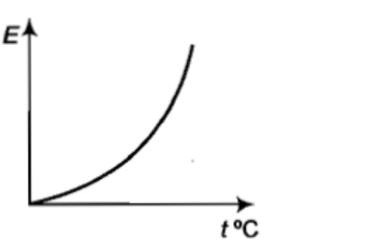An isolated system-

(A) is a specified region where transfers of energy and mass take place

(B) is a region of constant mass and only energy is allowed to close the boundaries

(C) is one in which mass within the system is not necessarily constant.

(D) cannot transfer either energy or mass to or from the surroundings.

Concept Questions :-

Ideal gas
NEET 2021 - Achiever Batch - Aryan Raj Singh

Difficulty Level:

The pressure and temperature of two different gases are P and T having the volume V for each.  They are mixed keeping the same volume and temperature, the pressure of the mixture will be-                                                          [Pb. PMT 1997, 98; DPMT 1999; MH CET 2003]

(1) P/2             (2) P             (3) 2P            (4) 4P

Concept Questions :-

Ideal gas
NEET 2021 - Achiever Batch - Aryan Raj Singh

Difficulty Level:

If the mean free path of atoms is doubled then the pressure of the gas will become:                                                                           [RPMT 2000]

(1) P/4            (2) P/2           (3) P/8           (4) P

Concept Questions :-

Mean free path
NEET 2021 - Achiever Batch - Aryan Raj Singh

Difficulty Level:

A diatomic molecule has how many degrees of freedom-                                                                                              [Pb. PET 2000]

(1) 3            (2) 4           (3) 5              (4) 6

Concept Questions :-

Law of equipartition of energy
NEET 2021 - Achiever Batch - Aryan Raj Singh

Difficulty Level:

Which of the following relations is correct for the root-mean-square speed of gas at temperature T?

Where, k= Boltzmann constant, and m= mass of a molecule.

(A)     (B)

(C)     (D)

Concept Questions :-

Types of velocity
NEET 2021 - Achiever Batch - Aryan Raj Singh

Difficulty Level:

A thermally insulated piston divides a container into two compartments.  Volume, temperature, and pressure in the right compartment are 2V, T, and 2P, while in the left compartment the respective values are V, T, and P.  If the piston can slide freely, then in the final equilibrium position, the volume of the right-hand compartment will be-

(1) V            (2) $\frac{3V}{5}$          (3) $\frac{9V}{4}$          (4) $\frac{12V}{5}$

Concept Questions :-

Ideal gas
NEET 2021 - Achiever Batch - Aryan Raj Singh

Difficulty Level:

The graph which represents the variation of mean kinetic energy of molecules with temperature $t°C$ is ?

(1)(2)(3)(4)Concept Questions :-

Kinetic energy of gas
NEET 2021 - Achiever Batch - Aryan Raj Singh

Difficulty Level:

${V}_{rms},{V}_{av},{V}_{mp}$ are root mean square, average and most probable speeds of molecules of a gas obeying Maxwellian velocity distribution. Which of the following statements is correct?

1. ${V}_{rms}<{V}_{av}<{V}_{mp}$

2. ${V}_{rms}>{V}_{av}>{V}_{mp}$

3.

4. ${V}_{avg}>{V}_{rms}<{V}_{mp}$

Concept Questions :-

Types of velocity
NEET 2021 - Achiever Batch - Aryan Raj Singh

Difficulty Level:

Gas is found to obey the law ${P}^{2}V$ = constant. The initial temperature and volume are ${T}_{0}$ and ${V}_{0}$. If the gas expands to a volume $3{V}_{0}$, its final temperature becomes:

(a) $\frac{{T}_{0}}{3}$           (b) $\frac{{T}_{0}}{\sqrt{3}}$

(c) $3{T}_{0}$           (d) None of these

Concept Questions :-

Ideal gas
NEET 2021 - Achiever Batch - Aryan Raj Singh

Difficulty Level:

For a gas, the r.m.s speed at 800 K is:

(1) Four times the value at 200 K

(2) Half the value at 200 K

(3) Twice the value at 200 K

(4) Same as at 200 K

Concept Questions :-

Types of velocity